Home > AC > Chapter 11 > Lesson 11.1.5 > Problem11-59

11-59.
1. Solve each equation below for the given variable. Be sure to check your solution. Homework Help ✎

1. 6x − 11 = 3x + 16

2. − 2(5 − 3x) + 5 = 9 + 3x

3.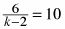4.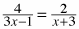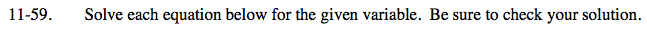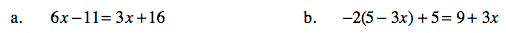Add 11 to both sides.

6x = 3x + 27

Subtract 3x from both sides.

3x = 27

Solve for x.

x = 9

Distribute the −2.

Follow the steps in part (a).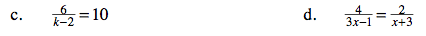Multiply both sides by a common factor of the denominators to remove the fractions.

6 = 10(k − 2)

Follow the steps in part (b).

Follow the steps in part (c).

x = 7